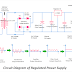Her, you can see the circuit diagram of regulated power supply. It is a power supply system that takes the unregulated alternating or AC power supply system as input and provides regulated direct or DC power supply as output. The main function of this system is to always keep constant the output voltage even when input voltage varies or connected load changes. The regulated power supply is very important and must require for sensitive electronic circuits, appliances, testing equipment. Generally, the output voltage of any normal power supply system changes or varies when the input of that power circuit changes or the load connected to that power circuit draws varies current. So the regulation is very important to get a stable or constant voltage. Anyway, first let's see the circuit diagram,

Here, you can see in the above regulated power supply circuit diagram, there are main four important parts of that system,
• Transformer
• Full Wave Bridge Rectifier Circuit
• Filter Circuit
• Regulator Circuit

Transformer

Here, we are talking about a 5V power supply system. So, the transformer used in this circuit is a step-down transformer and has a rating of 230V input AC supply and 6V output AC supply. So it converts the 230V AC supply into a 6V AC supply. The rating of the transformer depends upon the requirement of the output voltage of the circuit. If you want to make a 12V circuit, then you must take a transformer whose output is 12V.

Rectifier

The rectifier is a very important part of this circuit. It converts the 6V AC supply coming from the transformer output into 6V DC Supply. Here, you can see a full-wave bridge rectifier circuit is used for the rectification. The rectifier circuit has four PN Junction Diodes and each of them is rated IN 4007. Here, the diodes D2 and D4 convert the positive half cycle whereas the diodes D1 and D3 convert the negative half cycles. The full-wave bridge rectifier is the most efficient rectifier circuit as it converts both cycles. Also, it is a very cheaper and low-power losing device.

Filter Circuit

The output of the rectifier circuit is not pure. There are some AC components called ripple available in the output DC supply of the rectifier. So a filter circuit is required to filter the impure DC into pure DC. Here, in the above circuit diagram, a pure capacitor filter is used. You can see a 470 micro-farad, 50V electrolytic capacitor is used to filter the DC supply. In a DC power circuit, always an electrolytic capacitor should be used. The positive terminal of the capacitor is to be connected to the positive wire and the negative terminal of the capacitor is to be connected to the negative wire.

Regulator Circuit

It is the most important part of the regulated power supply circuit. Here, you can see the series voltage regulator IC 7805 is used. Here, the output voltage rating of the circuit is required 5V, that is why we used IC 7805. If want to make the circuit for 12V or 24V then you can use IC 7812 or IC 7824. Here, you can see also two ceramic capacitors rated 0.01 micro-farad are connected to the input and output of the regulator IC. This is for noise reduction and other different important factors.

Circuit Diagram of Regulated Power Supply with Component RatingReviewed by Author on 8:29 am Rating: 5